|

# 北京五一小长假首个潮玩盛会,就在北京国家会议中心

IJOY中国动漫游戏狂欢节 X CGF中国游戏节 X GPS国际潮流艺术展 三展联动汇集100+品牌，涵盖潮玩手办、时尚潮品、生活美学、博物馆文创冲限定，抢新发逛·玩·买，一次体验更多精彩活动尽在现场第一波展会品牌&展品公布↓↓↓《TOYCITY玩具城市》是一个以“帮助年轻人创造快乐，分享快乐为己任的年轻潮玩品牌。我们主张让每个人都能找到属于自己的小确幸的生活理念，通过搜罗全球优质IP资源、链接优秀设计师、对接高端品质生产厂家，为广大潮流爱好者打造丰富而有趣的“潮玩”产品。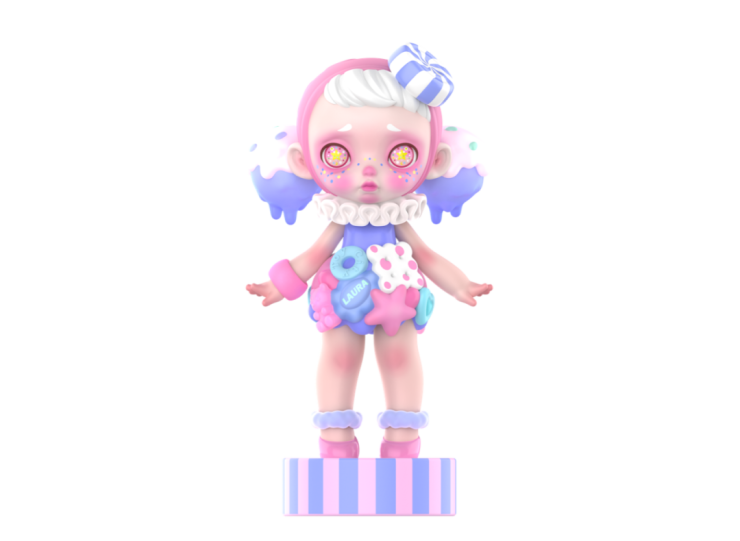LAURA 33cm展会限定款——莓果布丁、树莓布朗尼展会限定款1999元/体

LAURA展会限定款两款各299体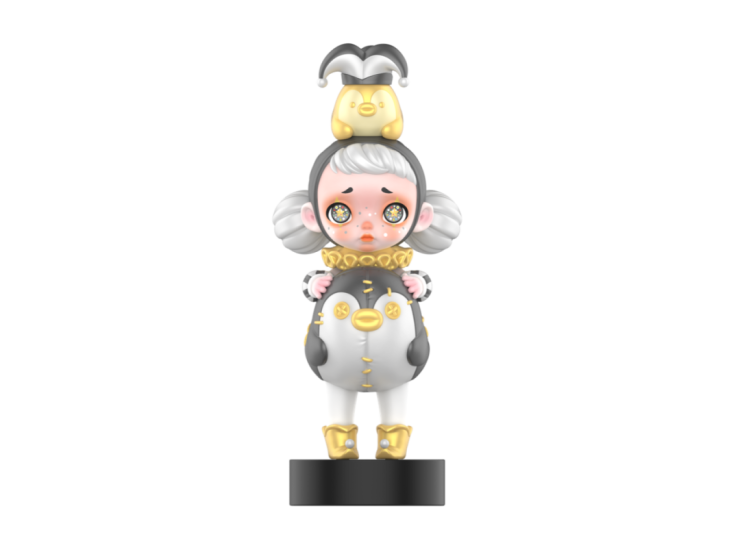LAURA×PUPU联名款——深夜PUPU、桃气PUPU、极光PUPU、星河PUPU联名款599/体

LAURA×PUPU联名款四款各499体来自露天马戏团的“星星眼少女Laura”，在探寻未知而美好生活的旅途上，在pupu planet遇到了一直寻找世上最美妙声音的PUPU，两个小可爱一拍即合，共同乘坐pupu Craft宇宙飞船前往宇宙的彼岸，寻找那未知的····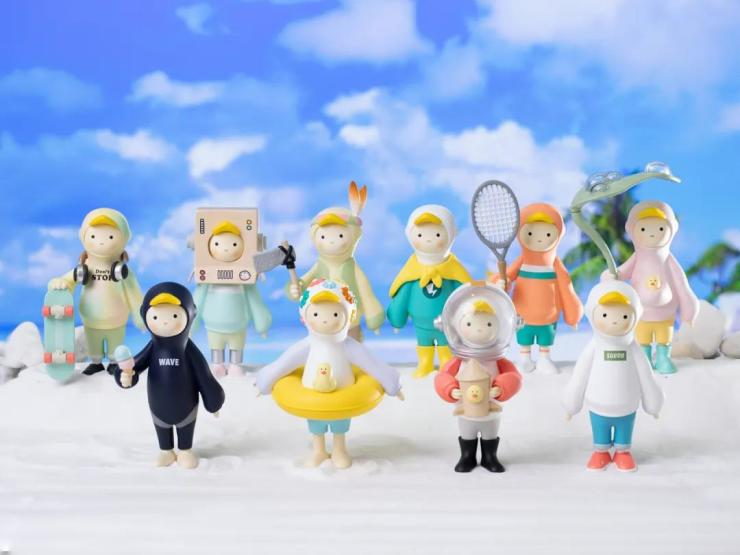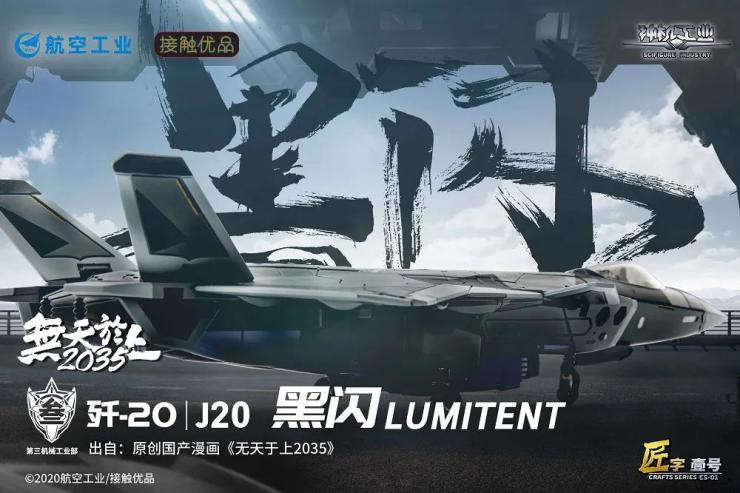《兔比比-珠光火龙果/珠光紫水晶》萌萌可爱的兔比比灵感源自于雕塑艺术家瞿广慈的经典作品“兔男郎”，双手托腮的兔比比可爱中又带着坏坏的淘气和色眯眯的浪漫情愫，他假装在睡觉可又没真正睡着，不过谁又能猜到他那鬼灵精怪的脑袋里装了哪些逗趣的点子呢？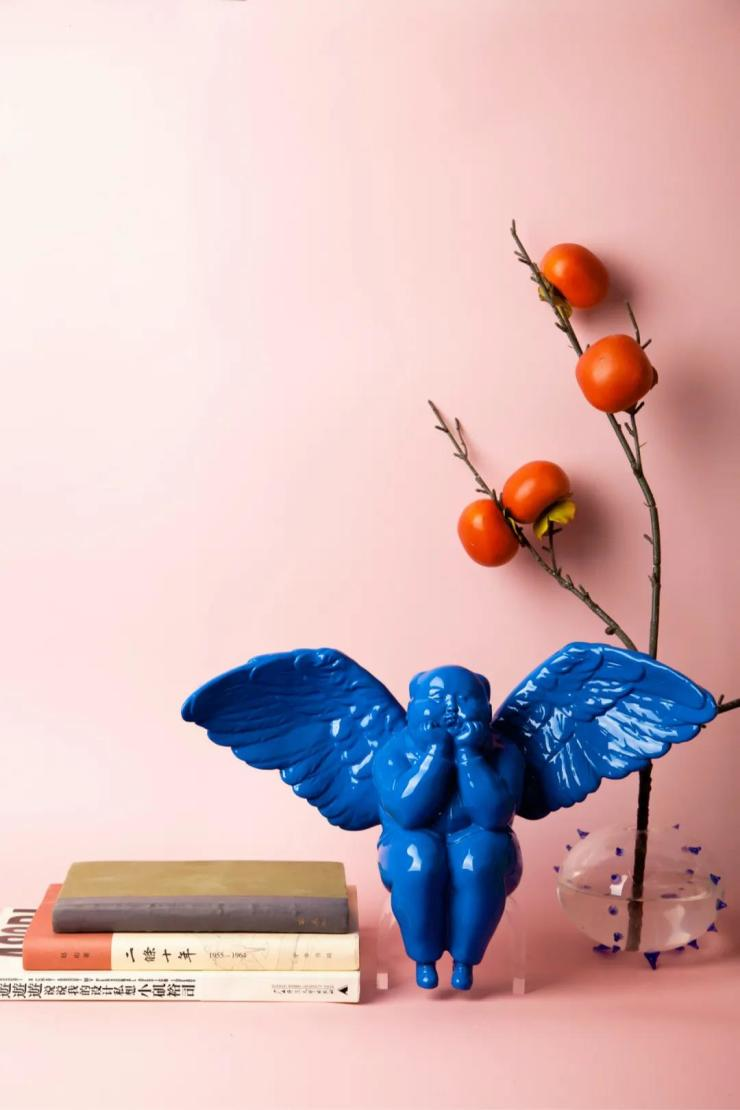《彩虹天使 · 虹》

25×12×36 cm（H*W*L）

7款配色：桃/白/橙/黄/绿/蓝/紫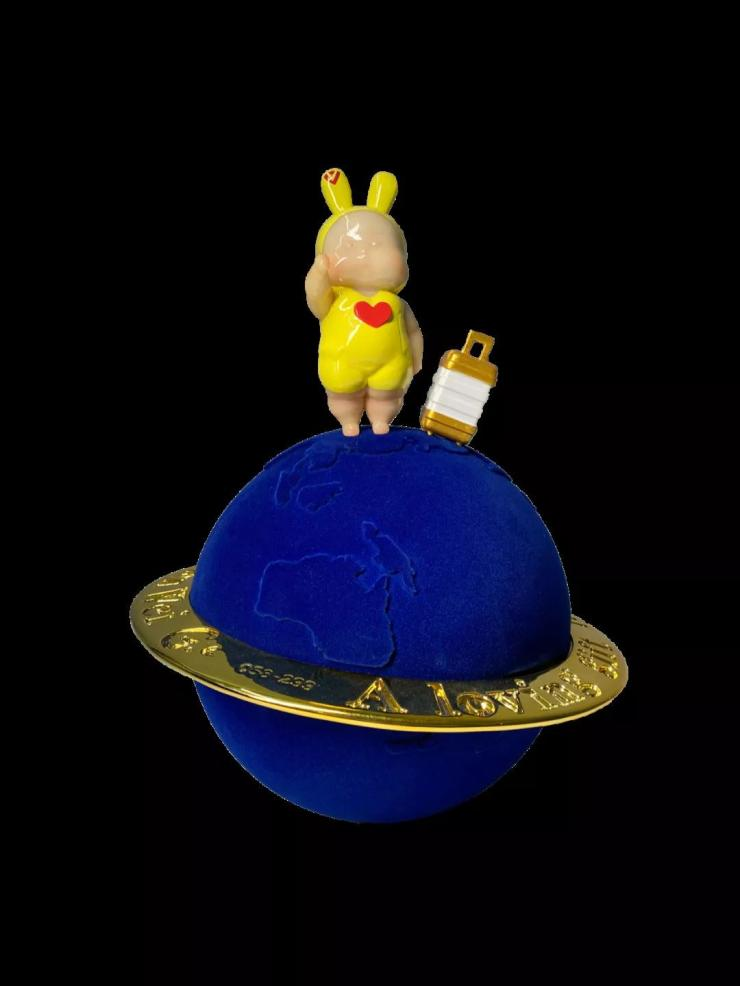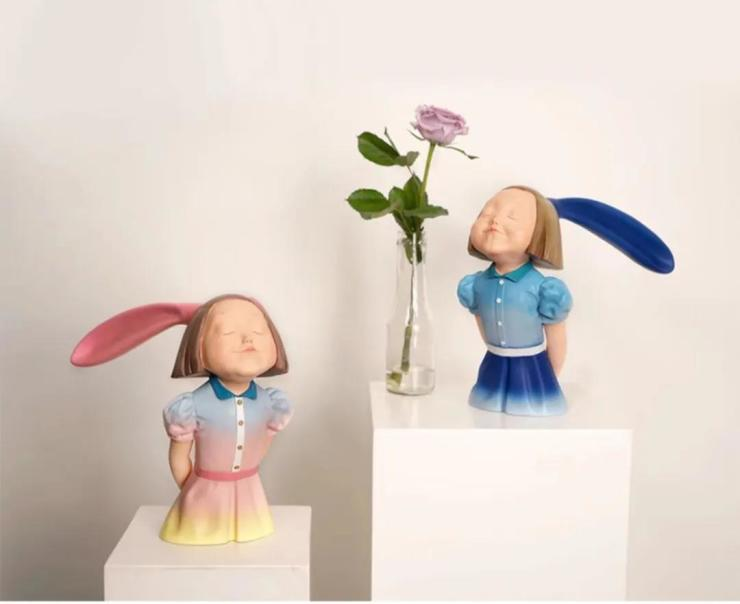《我看到了幸福-玫瑰湖畔/天空之境》BuBBle Fish 团队成员具备较高的造型综合能力与艺术创造能力，参与过很多领域的设计与创作，包括游戏原画、插画、动画片、影视分镜头等。致力打造高品质的潮流玩具。Little Demon ERIC虽然外表具备恶魔元素，但是真实想要表达的是萌娃成长过程中叛逆期的一种情绪，我们的ERIC是既可爱又淘气让人又爱又恨的萌娃。希望通过自己的努力给大家带来陪伴、开心和快乐。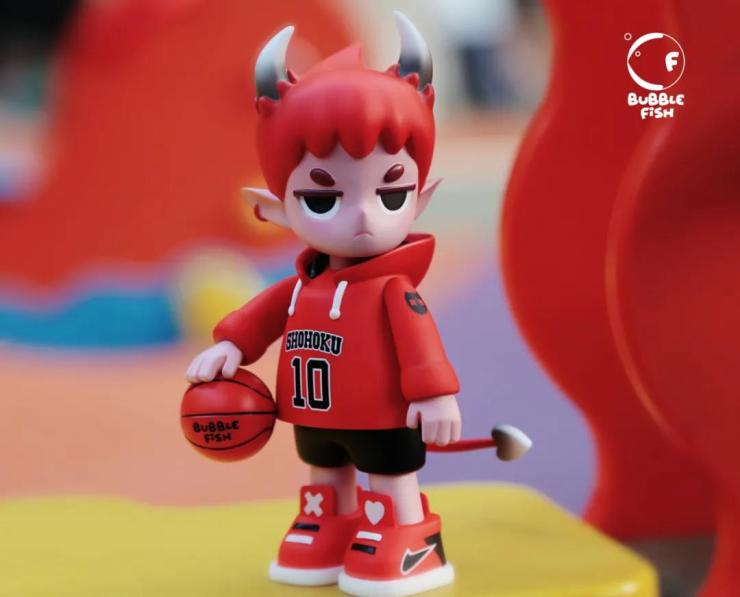“夏日の寄居蟹”盲盒  【新品】空想造物与设计师@大肚子jah联合出品，携手公益伙伴“红树林保育联盟”“守护荒野”聚焦滨海湿地生态系统保育。透明和实色两种材质，全套8只。“端坐猫”盲盒设计师不二马大叔，定格猫咪端坐凝视的一瞬间，因为逼真还原了真实的猫咪受到大家的喜爱。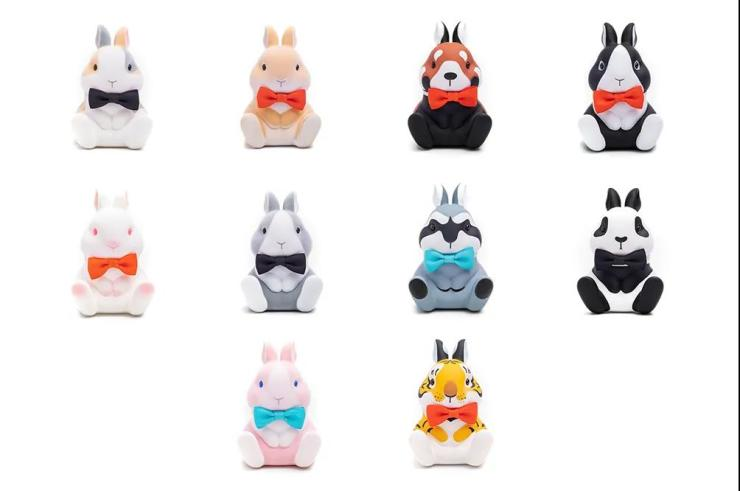“兔子”盲盒设计师不二马大叔，表面有植绒与手感漆两种效果，毛绒绒控和手感控都可以同时满足。植绒款花色根据真实宠物兔做艺术加工，满足大家对毛绒绒兔子的期望。手感漆款将其他一些人气很高的动物花色，例如大熊猫，小熊猫，老虎的花色与兔子的形象结合。“球球啾”盲盒原画师极危物种从世界范围选择极具特色的且人气很高的雀类创造了第2弹的肥啾形象，严格考究还原肥啾相应的特点和对应的鸟蛋纹路，极具科普意义。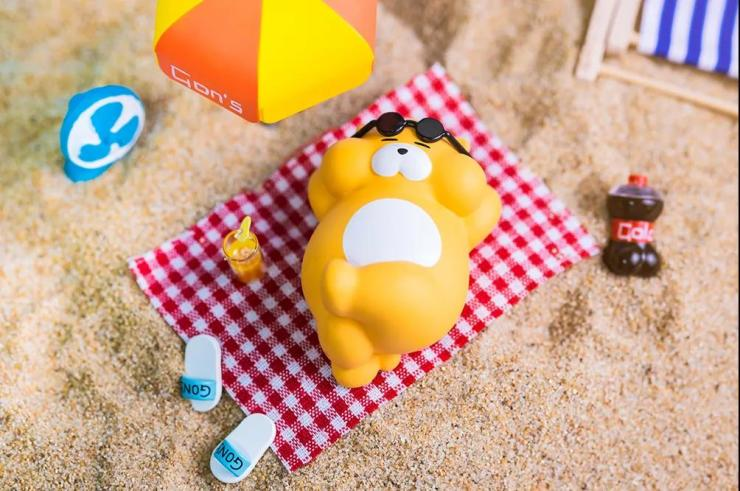“獭獭的日常”盲盒作者山药Gon，与十二栋合作，将大受欢迎的旱獭漫画人物制作成盲盒，粉丝基础庞大。全套9只，每只都配备趣味的小场景和配件。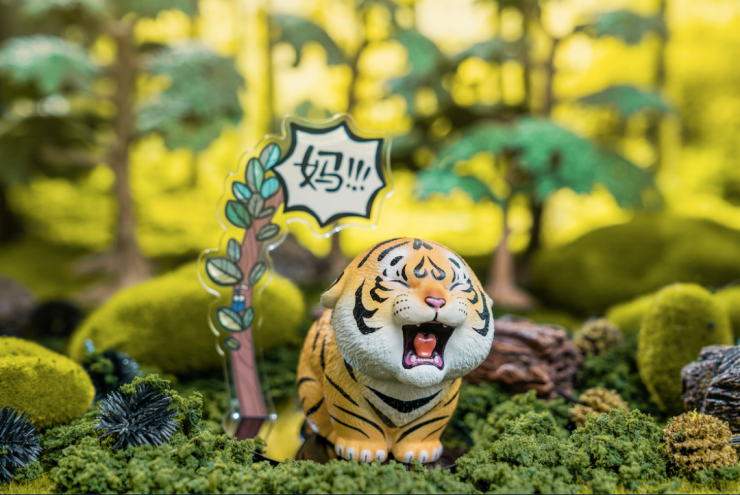“喊妈小虎”树脂雕像不二马大叔作品。“心有萌虎，再喊他妈。”仰着头张开嘴的小老虎仿佛定格住了喊妈的一瞬间，不经让我们想起了生活中的自己。“狮鹫”雕像设计师张凯渠，源自神话中的狮鹫的形象，融合肥啾与大猫的特点，塑造新的萌系狮鹫形象。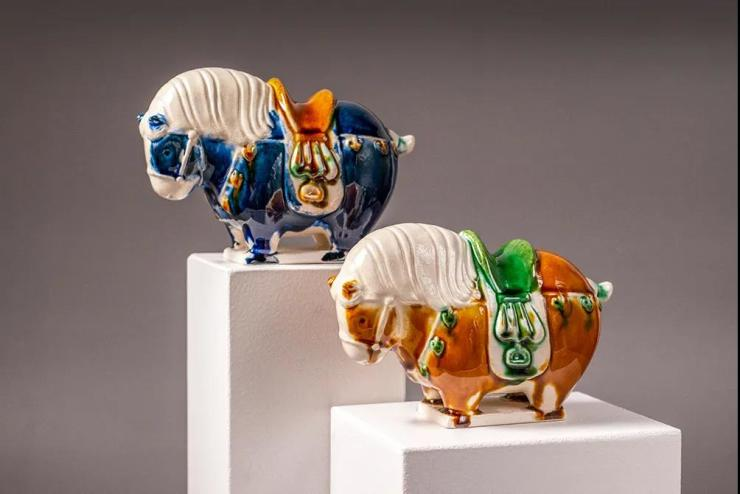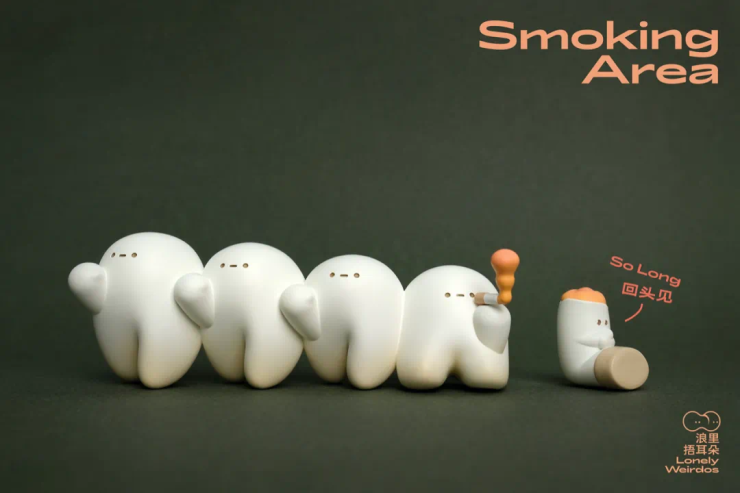SMOKING AREA吸烟区的居民是一群爱抽烟的小云，毕业后三只小云飞往别的城市，留下一只抽烟发呆。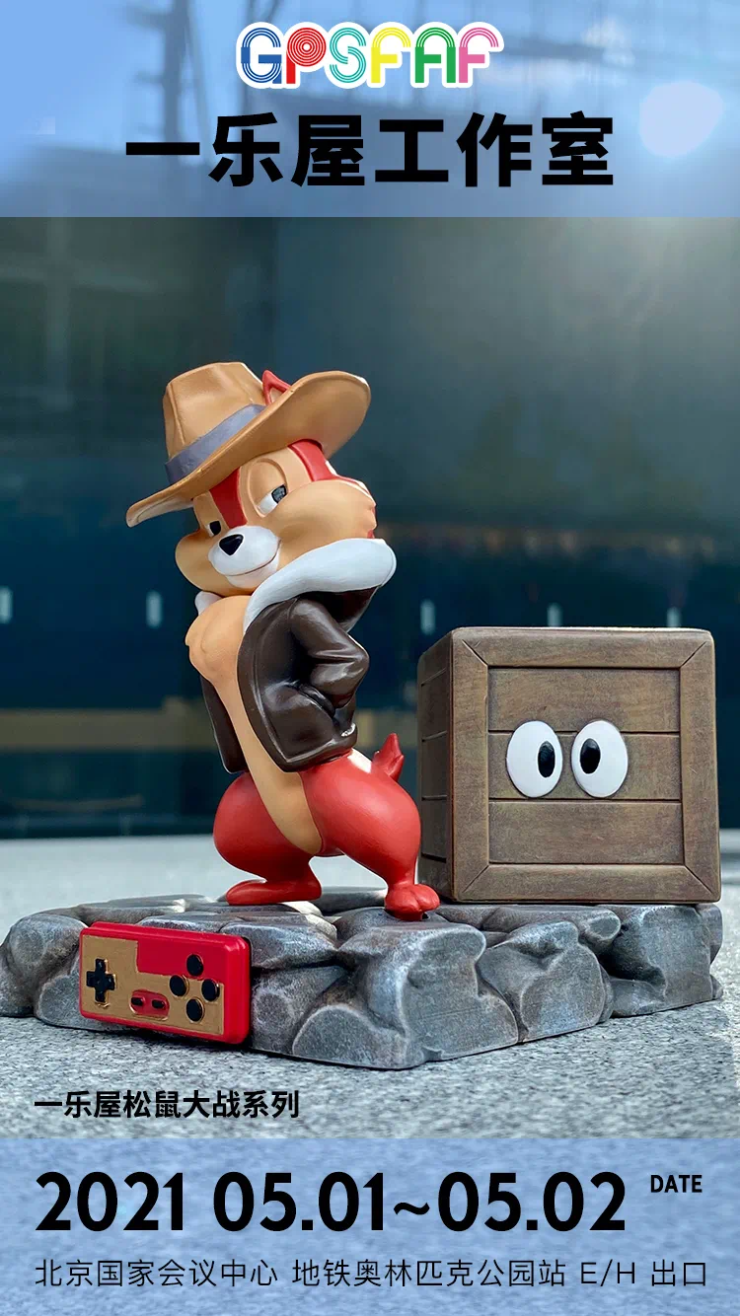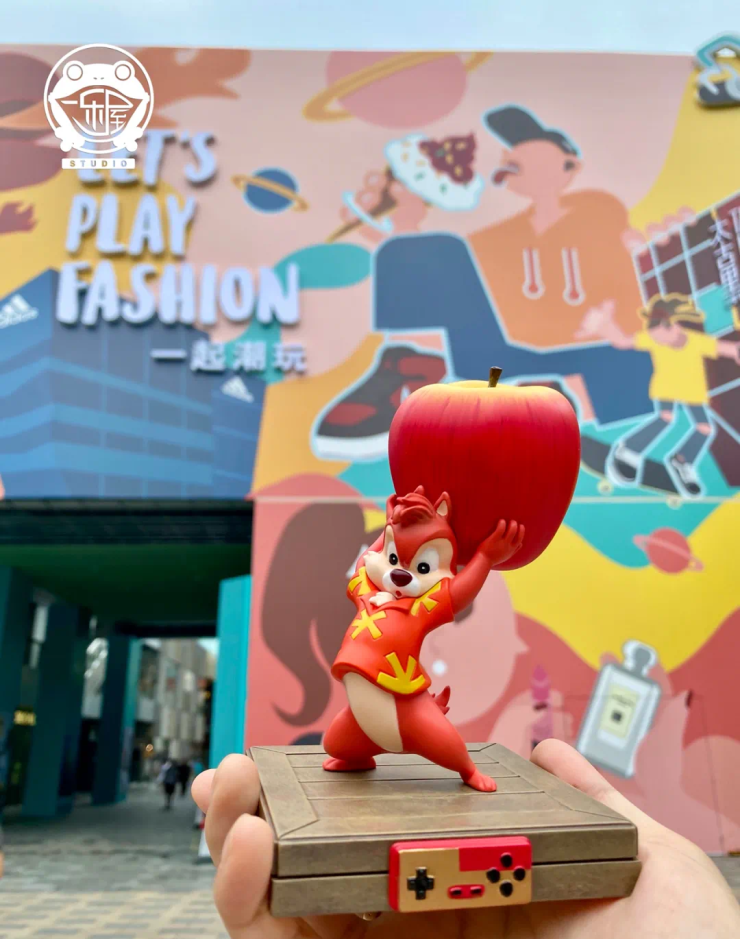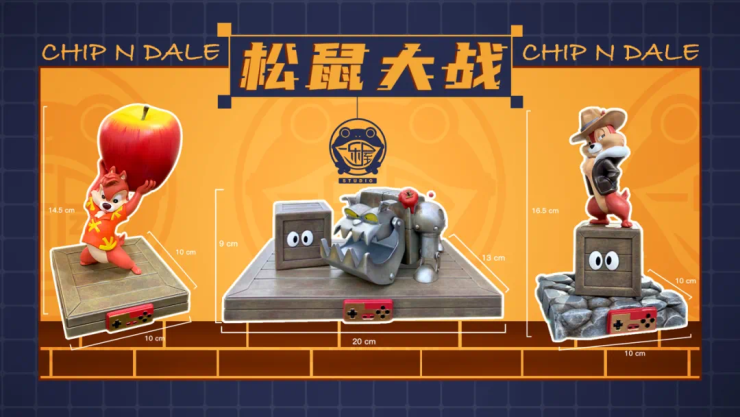1.奇奇站在蒂蒂躲藏的木箱下面

2.奇奇站在岩石地台上，一侧放着蒂蒂躲藏的木箱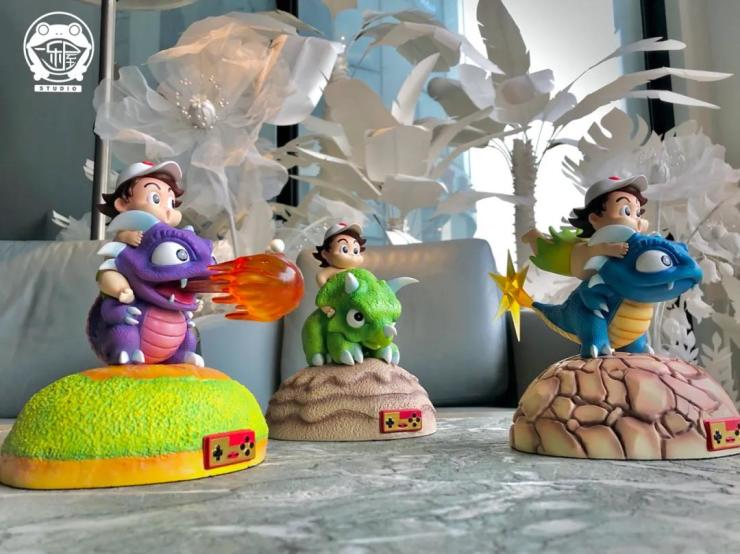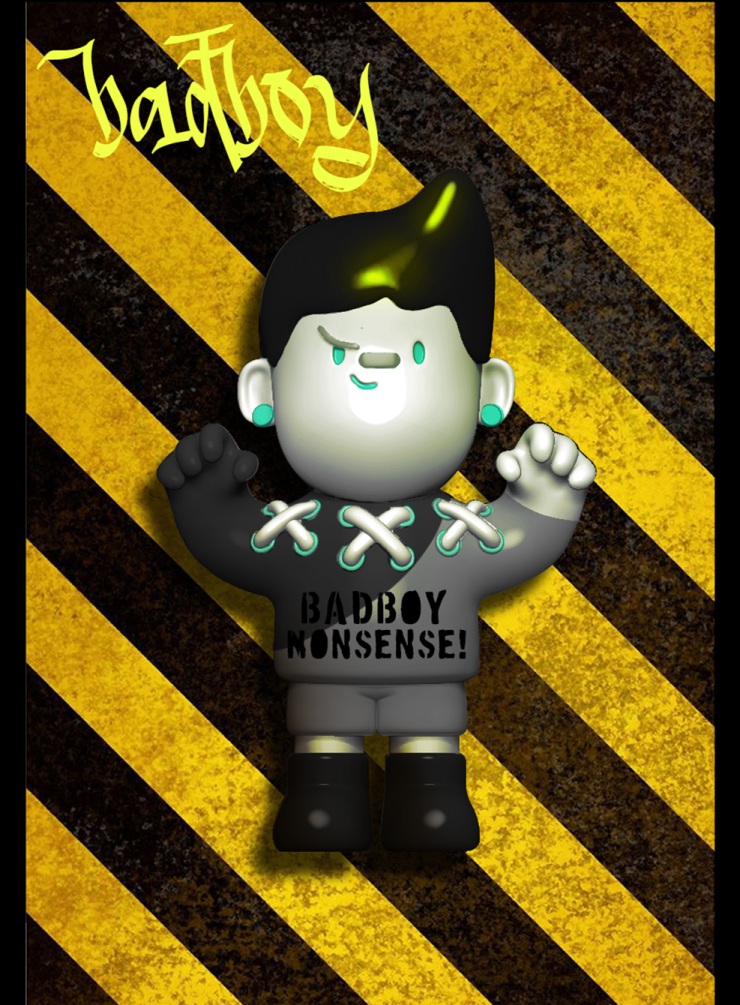MOMOplanet创立于2017年，活跃于艺术公仔、艺术潮流插画、雕塑等艺术潮流文化领域。MOMOplanet面世以来，迅速成为设计、潮流圈深受青睐的品牌之一。未来MOMOplanet将继续活跃于国内外艺术潮流领域，打造属于当下年轻人的卡通潮流IP形象，将“GO and Explore”的品牌理念贯穿于新一代潮流年轻人的生活方式中。PureArts Studio是享誉全球的收藏品制作商，拥有12年设计、开发和生产经验，致力于打造集艺术性和收藏性于一身的手办雕像作品。Daytoner (DTNR) 是Daniel Hahn正在创作的有关都市科幻题材的概念艺术项目，创作的重心是未来主义的机器人。Master 9 Eyes（M9E九眼大师）是PUREARTS®联名Daytoner首次推出设计师玩具项目。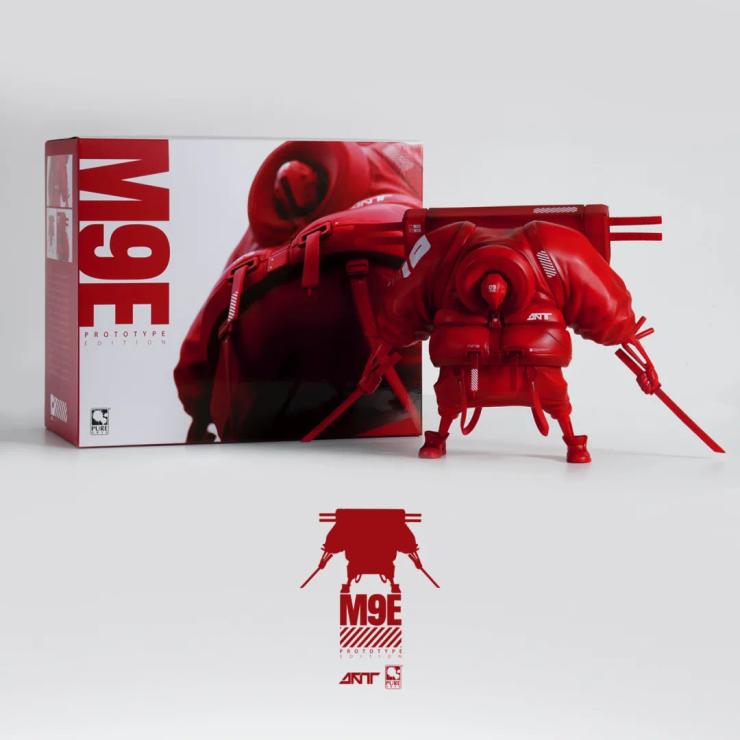M9E九眼大师  Original Red 原色3Q意为万物皆可爱·有趣（Q）。所谓道生一，一生二，二生三，三生万物，本品牌将取材于自然界中的万物，用艺术的语言让它们变的更加的有趣，以陶瓷创作的视角去塑造一个有趣的世界，为你带来一位新的玩具“朋友”。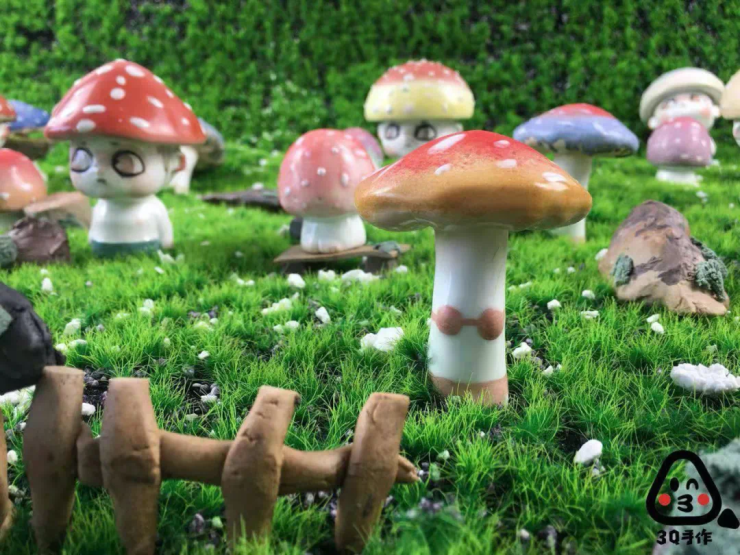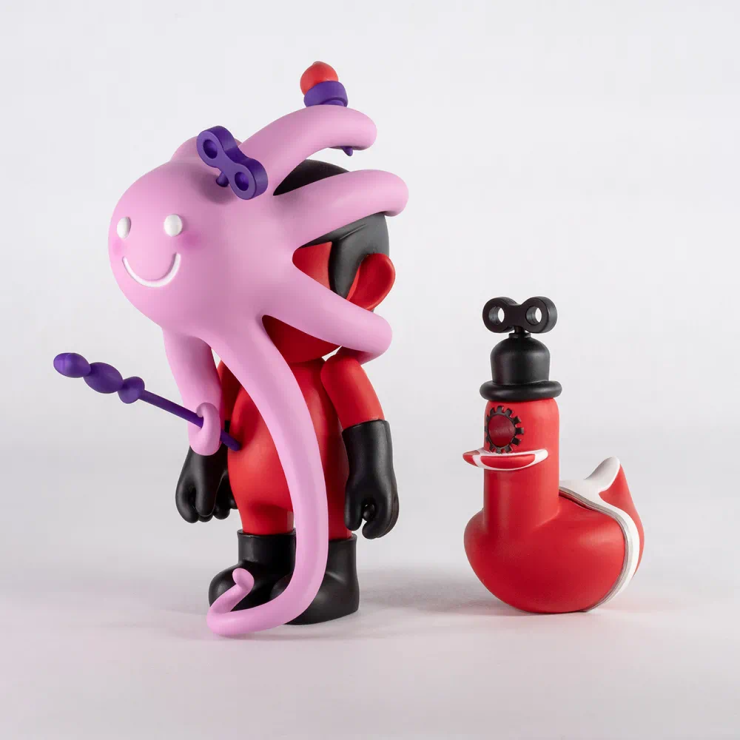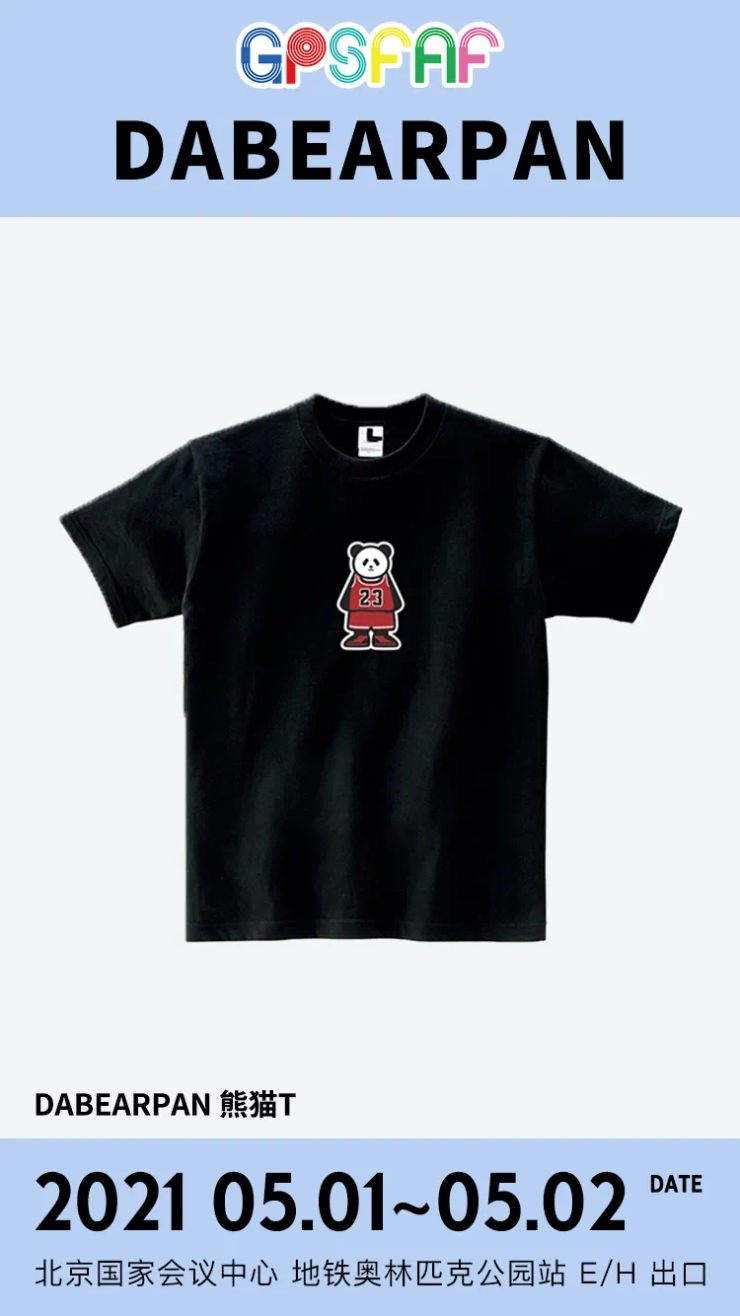“DABEARPAN"是国产潮牌“004”旗下的子品牌，以熊猫作为IP， 服装基调简约而慵懒，熊猫印花俏皮而可爱，整体风格为永不过时的街头风。在“DABEARPAN”的品牌背景中，设计出来的每一个熊猫都有着不同的性格，在生活中担当着不同的角色，就和如今的斜杠青年一样，可爱并且有着不同的才能，也具有着完全一致的生活习性——熬夜党，于是，我们都变成了可爱的熊猫。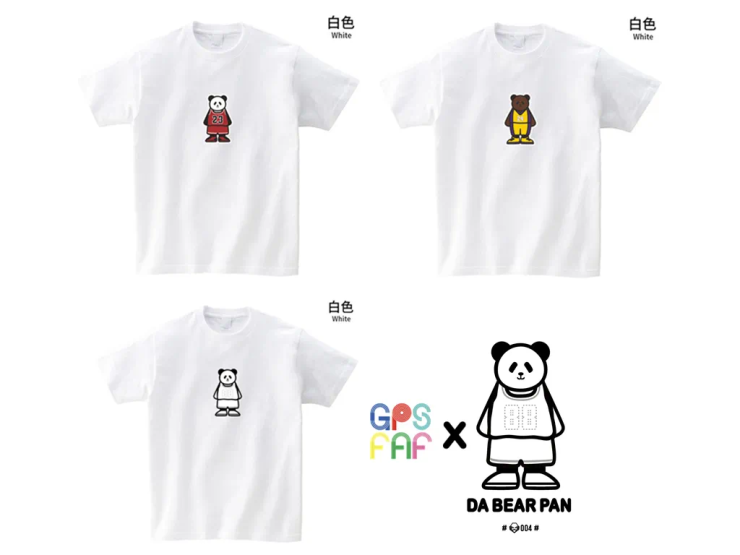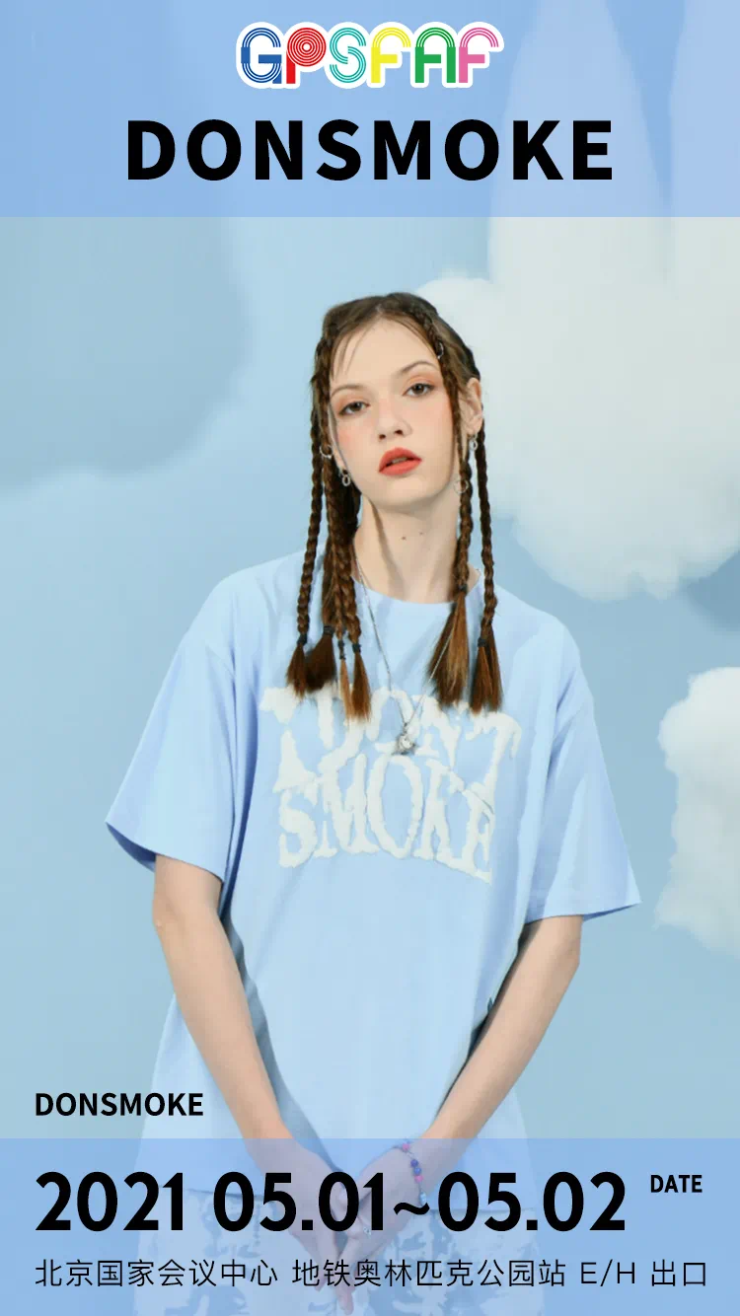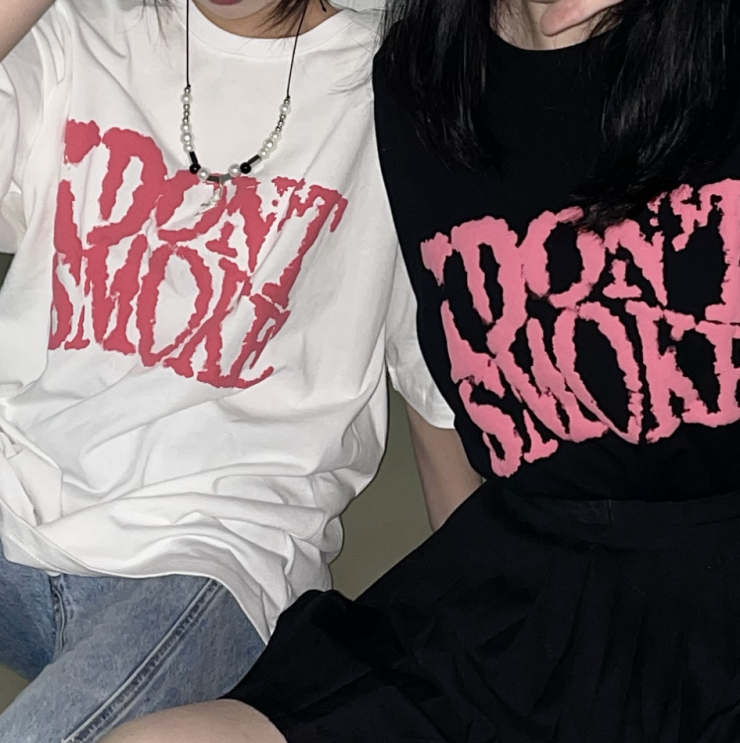DONSMOKE冬款卫衣的延续，将品牌SLOGAN「I DON’T SMOKE」变形印制胸前，使单品极具品牌辨识度，同时也准确的表达了品牌的文化理念和面对诱惑的态度——拒绝抽烟，在大家都抽烟的文化潮流圈下，不抽烟反而成了更特立独行的存在。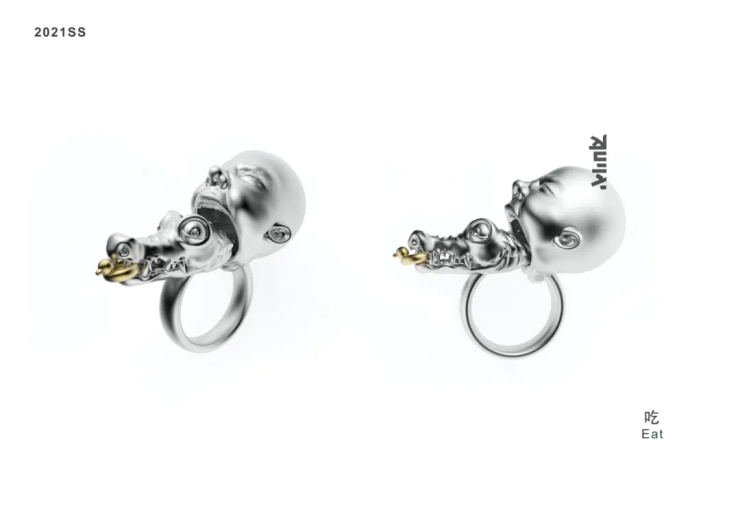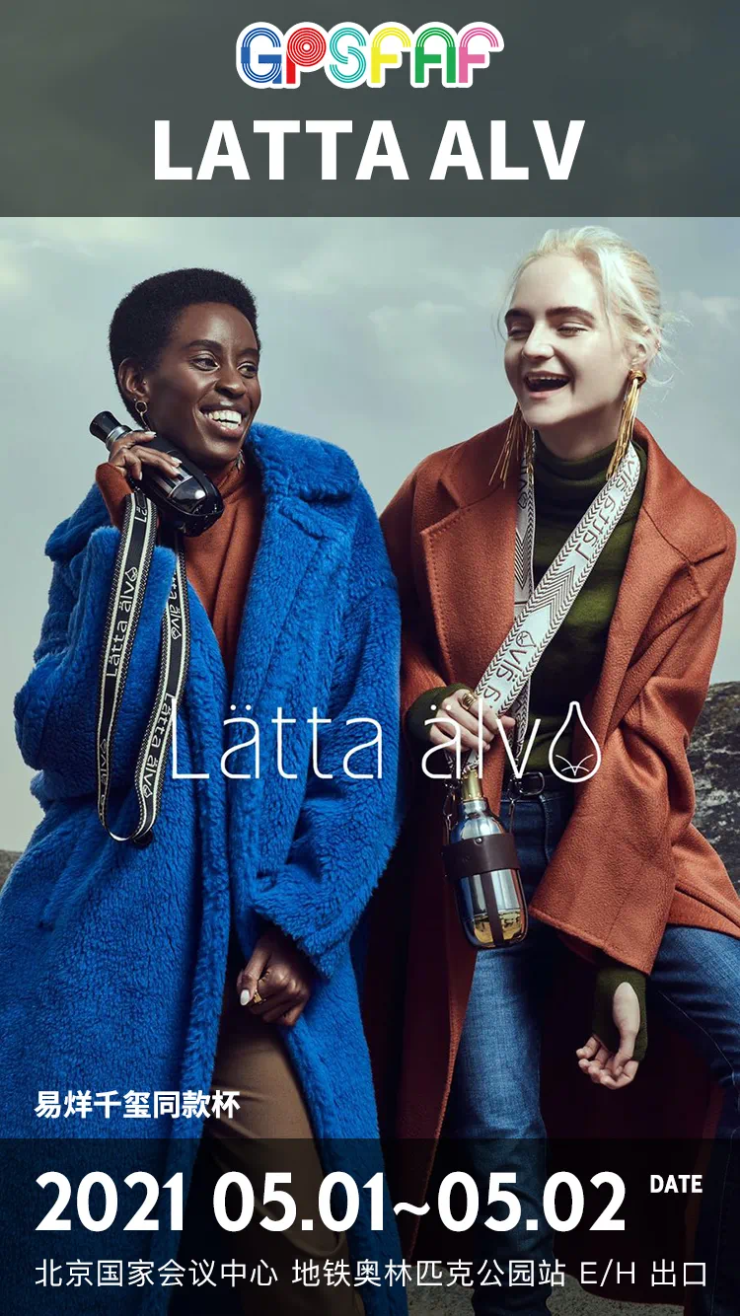LATTA ALV品牌设计师REZA SHAKORI，品牌理念：把一个杯子做到极致交给21世纪。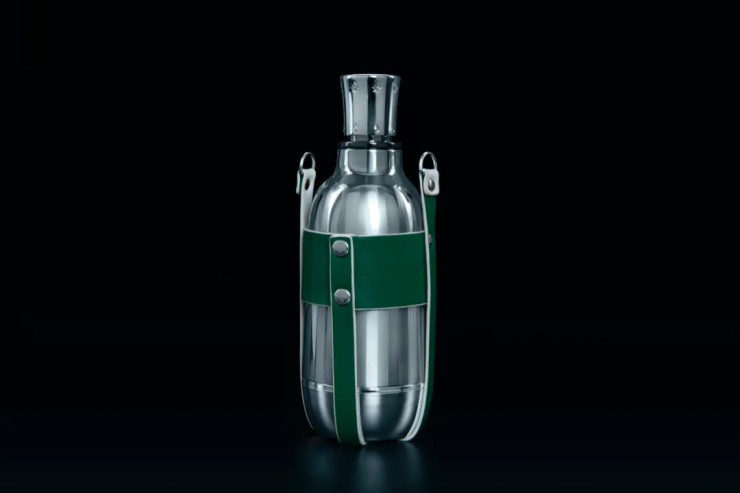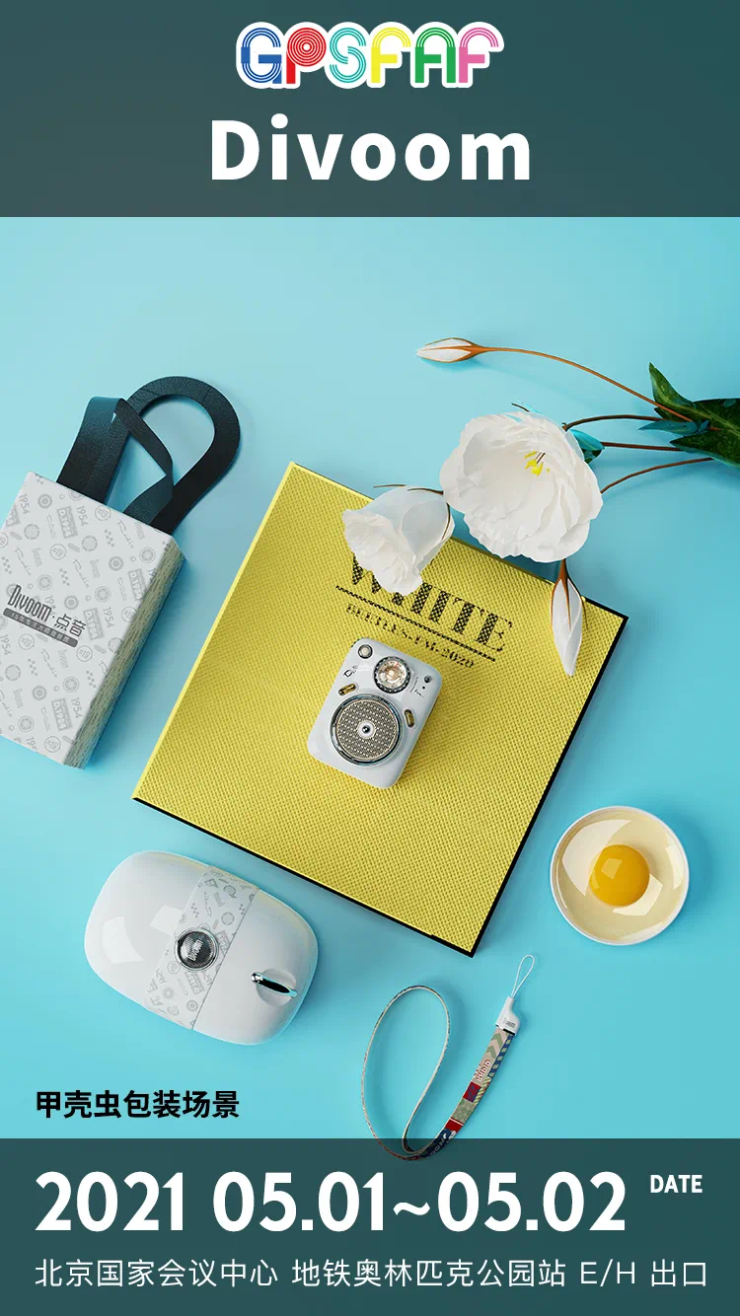Divoom点音2006年成立，专注于大师级音质15年，2015年发布全球第一款像素音箱。从成立之初就坚持国际化战略，至今，点音已经进入了全球60+个国家主流渠道。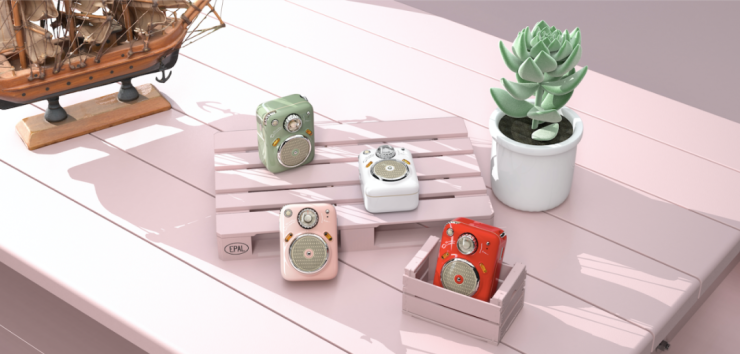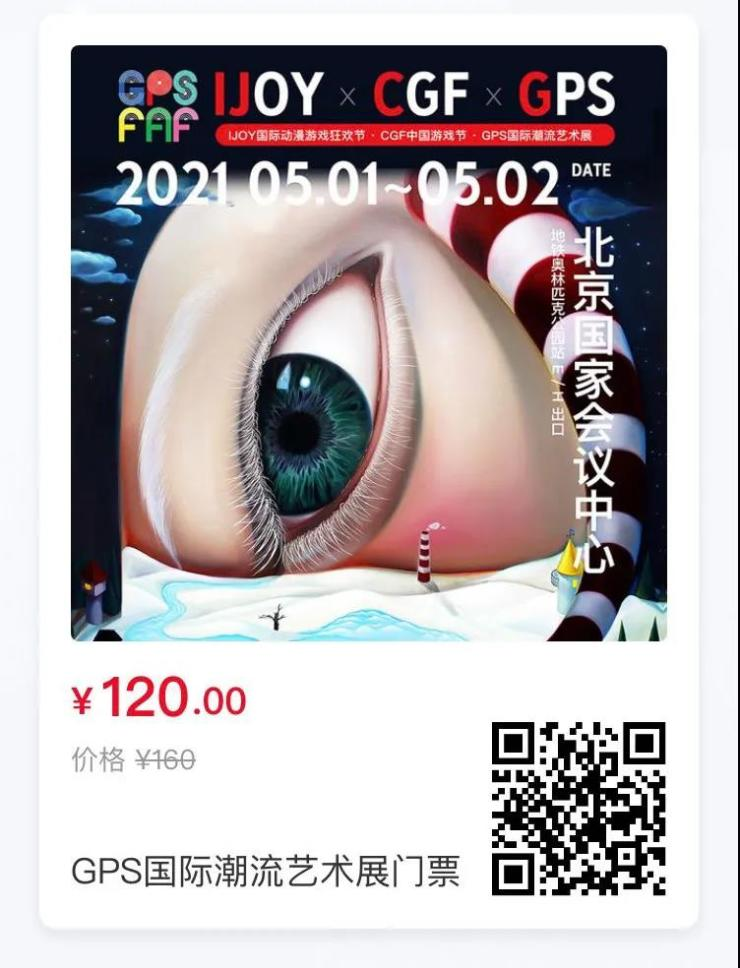`声明：本文由入驻焦点开放平台的作者撰写，除焦点官方账号外，观点仅代表作者本人，不代表焦点立场错误信息举报电话： 400-099-0099，邮箱：jubao@vip.sohu.com，或点此进行意见反馈，或点此进行举报投诉。`A B C D E F G H J K L M N P Q R S T W X Y Z
A - B - C - D - E
• A
• 鞍山
• 安庆
• 安阳
• 安顺
• 安康
• 澳门
• B
• 北京
• 保定
• 包头
• 巴彦淖尔
• 本溪
• 蚌埠
• 亳州
• 滨州
• 北海
• 百色
• 巴中
• 毕节
• 保山
• 宝鸡
• 白银
• 巴州
• C
• 承德
• 沧州
• 长治
• 赤峰
• 朝阳
• 长春
• 常州
• 滁州
• 池州
• 长沙
• 常德
• 郴州
• 潮州
• 崇左
• 重庆
• 成都
• 楚雄
• 昌都
• 慈溪
• 常熟
• D
• 大同
• 大连
• 丹东
• 大庆
• 东营
• 德州
• 东莞
• 德阳
• 达州
• 大理
• 德宏
• 定西
• 儋州
• 东平
• E
• 鄂尔多斯
• 鄂州
• 恩施
F - G - H - I - J
• F
• 抚顺
• 阜新
• 阜阳
• 福州
• 抚州
• 佛山
• 防城港
• G
• 赣州
• 广州
• 桂林
• 贵港
• 广元
• 广安
• 贵阳
• 固原
• H
• 邯郸
• 衡水
• 呼和浩特
• 呼伦贝尔
• 葫芦岛
• 哈尔滨
• 黑河
• 淮安
• 杭州
• 湖州
• 合肥
• 淮南
• 淮北
• 黄山
• 菏泽
• 鹤壁
• 黄石
• 黄冈
• 衡阳
• 怀化
• 惠州
• 河源
• 贺州
• 河池
• 海口
• 红河
• 汉中
• 海东
• 怀来
• I
• J
• 晋中
• 锦州
• 吉林
• 鸡西
• 佳木斯
• 嘉兴
• 金华
• 景德镇
• 九江
• 吉安
• 济南
• 济宁
• 焦作
• 荆门
• 荆州
• 江门
• 揭阳
• 金昌
• 酒泉
• 嘉峪关
K - L - M - N - P
• K
• 开封
• 昆明
• 昆山
• L
• 廊坊
• 临汾
• 辽阳
• 连云港
• 丽水
• 六安
• 龙岩
• 莱芜
• 临沂
• 聊城
• 洛阳
• 漯河
• 娄底
• 柳州
• 来宾
• 泸州
• 乐山
• 六盘水
• 丽江
• 临沧
• 拉萨
• 林芝
• 兰州
• 陇南
• M
• 牡丹江
• 马鞍山
• 茂名
• 梅州
• 绵阳
• 眉山
• N
• 南京
• 南通
• 宁波
• 南平
• 宁德
• 南昌
• 南阳
• 南宁
• 内江
• 南充
• P
• 盘锦
• 莆田
• 平顶山
• 濮阳
• 攀枝花
• 普洱
• 平凉
Q - R - S - T - W
• Q
• 秦皇岛
• 齐齐哈尔
• 衢州
• 泉州
• 青岛
• 清远
• 钦州
• 黔南
• 曲靖
• 庆阳
• R
• 日照
• 日喀则
• S
• 石家庄
• 沈阳
• 双鸭山
• 绥化
• 上海
• 苏州
• 宿迁
• 绍兴
• 宿州
• 三明
• 上饶
• 三门峡
• 商丘
• 十堰
• 随州
• 邵阳
• 韶关
• 深圳
• 汕头
• 汕尾
• 三亚
• 三沙
• 遂宁
• 山南
• 商洛
• 石嘴山
• T
• 天津
• 唐山
• 太原
• 通辽
• 铁岭
• 泰州
• 台州
• 铜陵
• 泰安
• 铜仁
• 铜川
• 天水
• 天门
• W
• 乌海
• 乌兰察布
• 无锡
• 温州
• 芜湖
• 潍坊
• 威海
• 武汉
• 梧州
• 渭南
• 武威
• 吴忠
• 乌鲁木齐
X - Y - Z
• X
• 邢台
• 徐州
• 宣城
• 厦门
• 新乡
• 许昌
• 信阳
• 襄阳
• 孝感
• 咸宁
• 湘潭
• 湘西
• 西双版纳
• 西安
• 咸阳
• 西宁
• 仙桃
• 西昌
• Y
• 运城
• 营口
• 盐城
• 扬州
• 鹰潭
• 宜春
• 烟台
• 宜昌
• 岳阳
• 益阳
• 永州
• 阳江
• 云浮
• 玉林
• 宜宾
• 雅安
• 玉溪
• 延安
• 榆林
• 银川
• Z
• 张家口
• 镇江
• 舟山
• 漳州
• 淄博
• 枣庄
• 郑州
• 周口
• 驻马店
• 株洲
• 张家界
• 珠海
• 湛江
• 肇庆
• 中山
• 自贡
• 资阳
• 遵义
• 昭通
• 张掖
• 中卫

1室1厅1厨1卫1阳台

1
2
3
4
5

0
1
2

1

1

0
1
2
3报名成功，资料已提交审核A B C D E F G H J K L M N P Q R S T W X Y Z
A - B - C - D - E
• A
• 鞍山
• 安庆
• 安阳
• 安顺
• 安康
• 澳门
• B
• 北京
• 保定
• 包头
• 巴彦淖尔
• 本溪
• 蚌埠
• 亳州
• 滨州
• 北海
• 百色
• 巴中
• 毕节
• 保山
• 宝鸡
• 白银
• 巴州
• C
• 承德
• 沧州
• 长治
• 赤峰
• 朝阳
• 长春
• 常州
• 滁州
• 池州
• 长沙
• 常德
• 郴州
• 潮州
• 崇左
• 重庆
• 成都
• 楚雄
• 昌都
• 慈溪
• 常熟
• D
• 大同
• 大连
• 丹东
• 大庆
• 东营
• 德州
• 东莞
• 德阳
• 达州
• 大理
• 德宏
• 定西
• 儋州
• 东平
• E
• 鄂尔多斯
• 鄂州
• 恩施
F - G - H - I - J
• F
• 抚顺
• 阜新
• 阜阳
• 福州
• 抚州
• 佛山
• 防城港
• G
• 赣州
• 广州
• 桂林
• 贵港
• 广元
• 广安
• 贵阳
• 固原
• H
• 邯郸
• 衡水
• 呼和浩特
• 呼伦贝尔
• 葫芦岛
• 哈尔滨
• 黑河
• 淮安
• 杭州
• 湖州
• 合肥
• 淮南
• 淮北
• 黄山
• 菏泽
• 鹤壁
• 黄石
• 黄冈
• 衡阳
• 怀化
• 惠州
• 河源
• 贺州
• 河池
• 海口
• 红河
• 汉中
• 海东
• 怀来
• I
• J
• 晋中
• 锦州
• 吉林
• 鸡西
• 佳木斯
• 嘉兴
• 金华
• 景德镇
• 九江
• 吉安
• 济南
• 济宁
• 焦作
• 荆门
• 荆州
• 江门
• 揭阳
• 金昌
• 酒泉
• 嘉峪关
K - L - M - N - P
• K
• 开封
• 昆明
• 昆山
• L
• 廊坊
• 临汾
• 辽阳
• 连云港
• 丽水
• 六安
• 龙岩
• 莱芜
• 临沂
• 聊城
• 洛阳
• 漯河
• 娄底
• 柳州
• 来宾
• 泸州
• 乐山
• 六盘水
• 丽江
• 临沧
• 拉萨
• 林芝
• 兰州
• 陇南
• M
• 牡丹江
• 马鞍山
• 茂名
• 梅州
• 绵阳
• 眉山
• N
• 南京
• 南通
• 宁波
• 南平
• 宁德
• 南昌
• 南阳
• 南宁
• 内江
• 南充
• P
• 盘锦
• 莆田
• 平顶山
• 濮阳
• 攀枝花
• 普洱
• 平凉
Q - R - S - T - W
• Q
• 秦皇岛
• 齐齐哈尔
• 衢州
• 泉州
• 青岛
• 清远
• 钦州
• 黔南
• 曲靖
• 庆阳
• R
• 日照
• 日喀则
• S
• 石家庄
• 沈阳
• 双鸭山
• 绥化
• 上海
• 苏州
• 宿迁
• 绍兴
• 宿州
• 三明
• 上饶
• 三门峡
• 商丘
• 十堰
• 随州
• 邵阳
• 韶关
• 深圳
• 汕头
• 汕尾
• 三亚
• 三沙
• 遂宁
• 山南
• 商洛
• 石嘴山
• T
• 天津
• 唐山
• 太原
• 通辽
• 铁岭
• 泰州
• 台州
• 铜陵
• 泰安
• 铜仁
• 铜川
• 天水
• 天门
• W
• 乌海
• 乌兰察布
• 无锡
• 温州
• 芜湖
• 潍坊
• 威海
• 武汉
• 梧州
• 渭南
• 武威
• 吴忠
• 乌鲁木齐
X - Y - Z
• X
• 邢台
• 徐州
• 宣城
• 厦门
• 新乡
• 许昌
• 信阳
• 襄阳
• 孝感
• 咸宁
• 湘潭
• 湘西
• 西双版纳
• 西安
• 咸阳
• 西宁
• 仙桃
• 西昌
• Y
• 运城
• 营口
• 盐城
• 扬州
• 鹰潭
• 宜春
• 烟台
• 宜昌
• 岳阳
• 益阳
• 永州
• 阳江
• 云浮
• 玉林
• 宜宾
• 雅安
• 玉溪
• 延安
• 榆林
• 银川
• Z
• 张家口
• 镇江
• 舟山
• 漳州
• 淄博
• 枣庄
• 郑州
• 周口
• 驻马店
• 株洲
• 张家界
• 珠海
• 湛江
• 肇庆
• 中山
• 自贡
• 资阳
• 遵义
• 昭通
• 张掖
• 中卫• 手机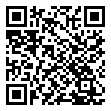• 分享
• 设计
免费设计
• 计算器
装修计算器
• 入驻
合作入驻
• 联系
联系我们
• 置顶
返回顶部